# Back to Assignment Attempts: 30 Keep the Highest: 3/4 . The cost of retained earnings The cost of...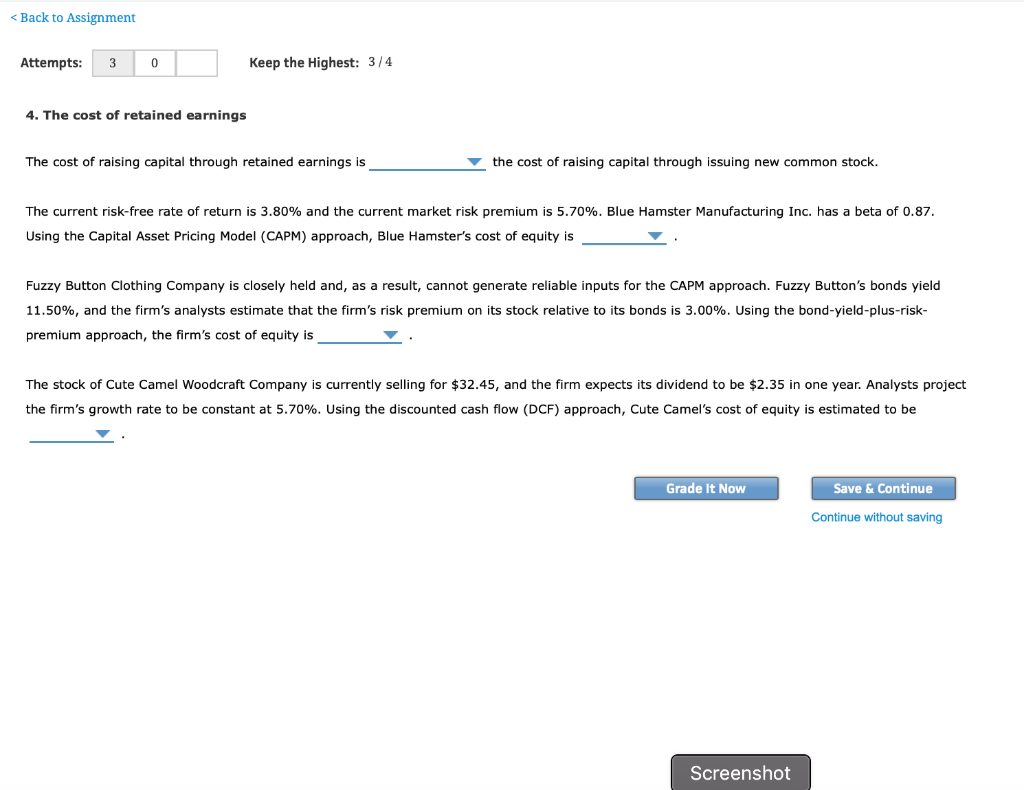Back to Assignment Attempts: 30 Keep the Highest: 3/4 . The cost of retained earnings The cost of raising capital through retained earnings is the cost of raising capital through issuing new common stock. The current risk-free rate of return is 3.80% and the current market risk premium is 5.70%. Blue Hamster Manufacturing Inc. has a beta of 0.87. Using the Capital Asset Pricing Model (CAPM) approach, Blue Hamster's cost of equity is Fuzzy Button Clothing Company is closely held and, as a result, cannot generate reliable inputs for the CAPM approach. Fuzzy Button's bonds yield 11.50%, and the firm's analysts estimate that the firm's risk premium on its stock relative to its bonds is 3.00%. Using the bond-yield-plus-risk- premium approach, the firm's cost of equity is The stock of Cute Camel Woodcraft Company is currently selling for \$32.45, and the firm expects its dividend to be \$2.35 in one year. Analysts project the firm's growth rate to be constant at 5.70%. Using the discounted cash flow (DCF) approach, Cute Camel's cost of equity is estimated to be Grade It Now Save & Continue Continue without saving Screenshot

a) The cost of raising capital through retained earning is less costlier /less expensive than cost of raising through issuing new equity

B) cost of equity =risk free rate+(beta*market risk premium)

= 3.8 + (.87*5.7)

=3.8+ 4.96

= 8.76%

C) cost of equity =Bond yield +risk premium

= 11.5 +3

= 14.5%

D) cost of equity=(D1/price)+growth

(2.35/32.45) +.057

.0724 +.057

.1294 or 12.94%

##### Add Answer of: Back to Assignment Attempts: 30 Keep the Highest: 3/4 . The cost of retained earnings The cost of...
Similar Homework Help Questions
• ### The cost of raising capital through retained earnings is new common stock. the cost of raising...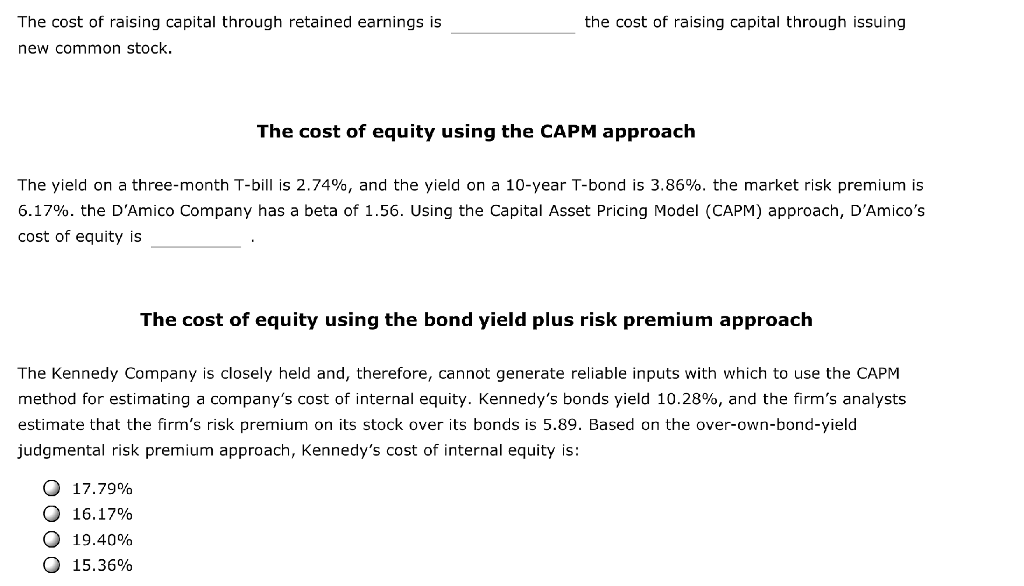The cost of raising capital through retained earnings is new common stock. the cost of raising capital through issuing The cost of equity using the CAPM approach The yield on a three-month T-bill is 2.74%, and the yield on a 10-year T-bond is 3.86%, the market risk premium is 6.17%, the D'Amico Company has a beta of 1.56. Using the Capital Asset Pricing Model (CAPM) approach, DAmico's cost of equity is The cost of equity using the bond yield plus...

• ### The cost of raising capital through retained earnings is the cost of raising capital through issuing new common stock....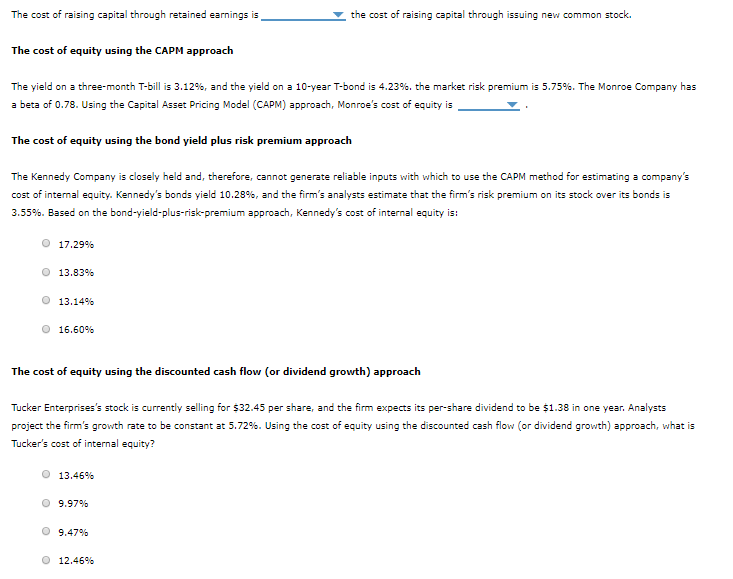The cost of raising capital through retained earnings is the cost of raising capital through issuing new common stock. The cost of equity using the CAPM approach The yield on a three-month T-bill is 3.12%, and the yield on a 10-year T-bond is 4.23%, the market risk premium is 5.75%. The Monroe Company has a beta of 0.78. Using the Capital Asset Pricing Model (CAPM) approach, Monroe's cost of equity is The cost of equity using the bond yield plus...

• ### 4. The cost of retained earnings The cost of raising capital through retained earnings is   the...

4. The cost of retained earnings The cost of raising capital through retained earnings is   the cost of raising capital through issuing new common stock. The cost of equity using the CAPM approach The current risk-free rate of return (rRFrRF) is 3.86% while the market risk premium is 6.17%. The Burris Company has a beta of 0.92. Using the capital asset pricing model (CAPM) approach, Burris’s cost of equity is     . The cost of equity using the bond yield plus...

• ### Attempts: Average: 15 S. The cost of retained earnings The cost of retained earnings The cost...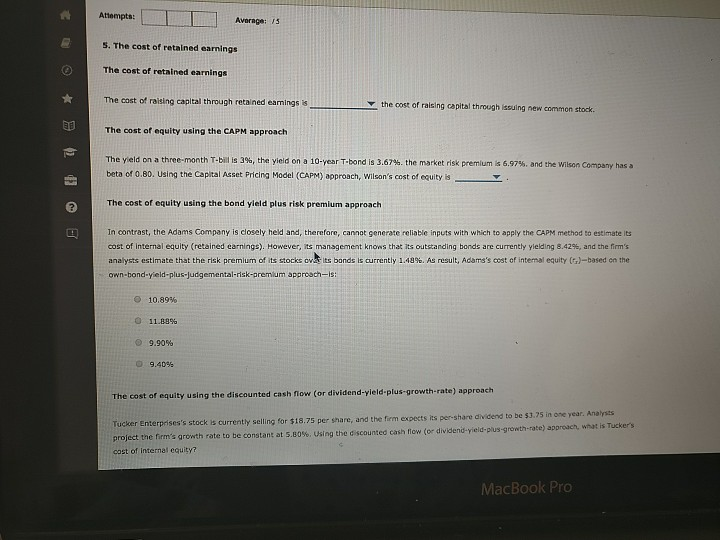Attempts: Average: 15 S. The cost of retained earnings The cost of retained earnings The cost of raising capital through retained earings is the cost of raising capital through issuing new common stock. The cost of equity using the CAPM approach The yield on a three-month T-bills 39, the yield on a 10-year T-bond is 3.67%, the market risk premium beta of 0.B0. Using the Capital Asset Pricing Model (CAPM) approach, Wilson's cost of equity is 6.97% and the Wilson...

• ### 6. The cost of retained earnings Aa Aa E the cost of raising capital through issuing...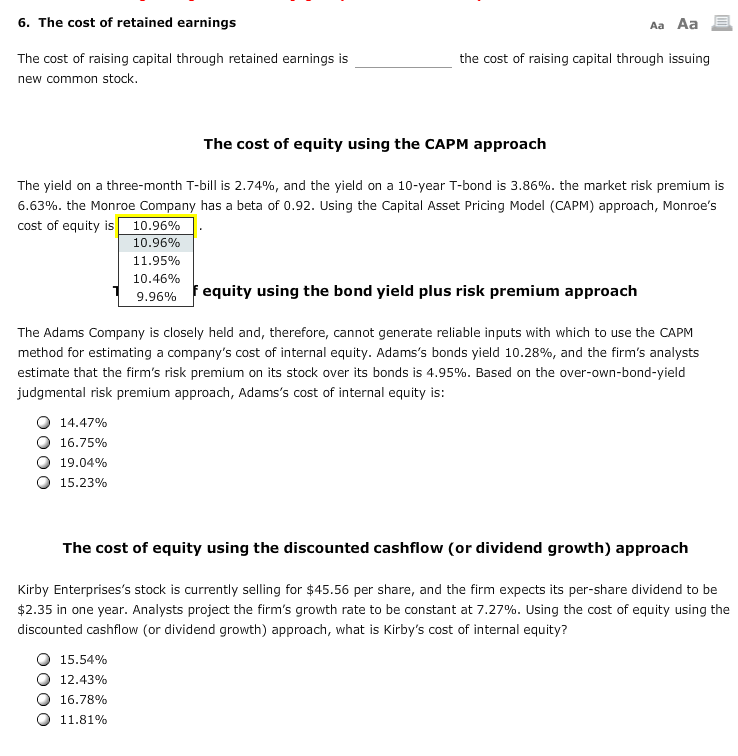6. The cost of retained earnings Aa Aa E the cost of raising capital through issuing The cost of raising capital through retained earnings is new common stock. The cost of equity using the CAPM approach The yield on a three-month T-bill is 2.74%, and the yield on a 10-year T-bond is 3.86%. the market risk premium is 6.63%. the Monroe Company has a beta of 0.92. Using the Capital Asset Pricing Model (CAPM) approach, Monroe's cost of equity is...

• ### 5. The cost of retained earnings Aa Aa E the cost of raising capital through issuing...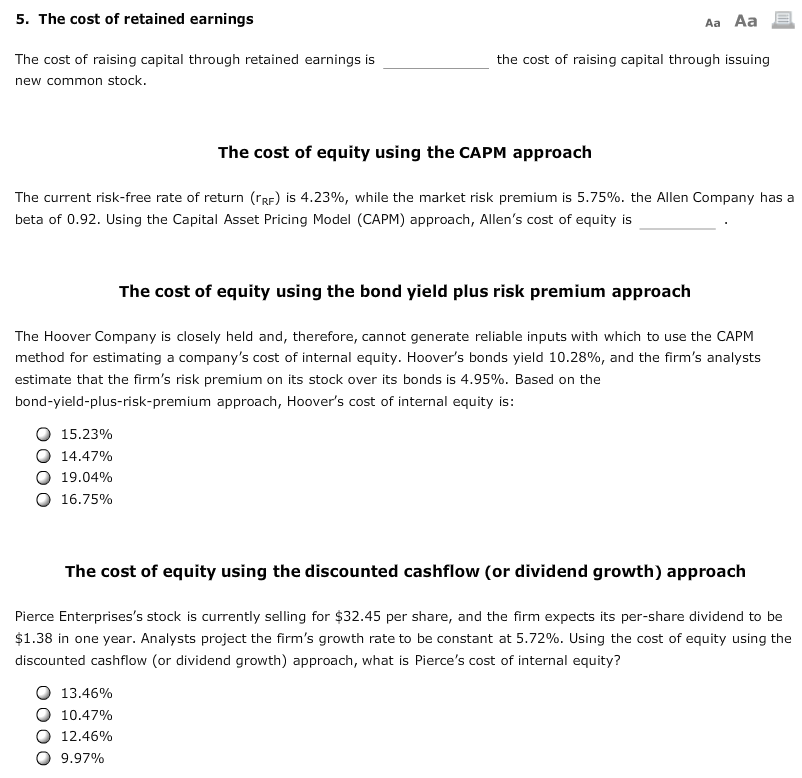5. The cost of retained earnings Aa Aa E the cost of raising capital through issuing The cost of raising capital through retained earnings is new common stock. The cost of equity using the CAPM approach The current risk-free rate of return (PRF) is 4.23%, while the market risk premium is 5.75%. the Allen Company has a beta of 0.92. Using the Capital Asset Pricing Model (CAPM) approach, Allen's cost of equity is The cost of equity using the bond...

• ### 4. The cost of retained earnings True or False: It is free for a company to raise money through retained earnings, beca...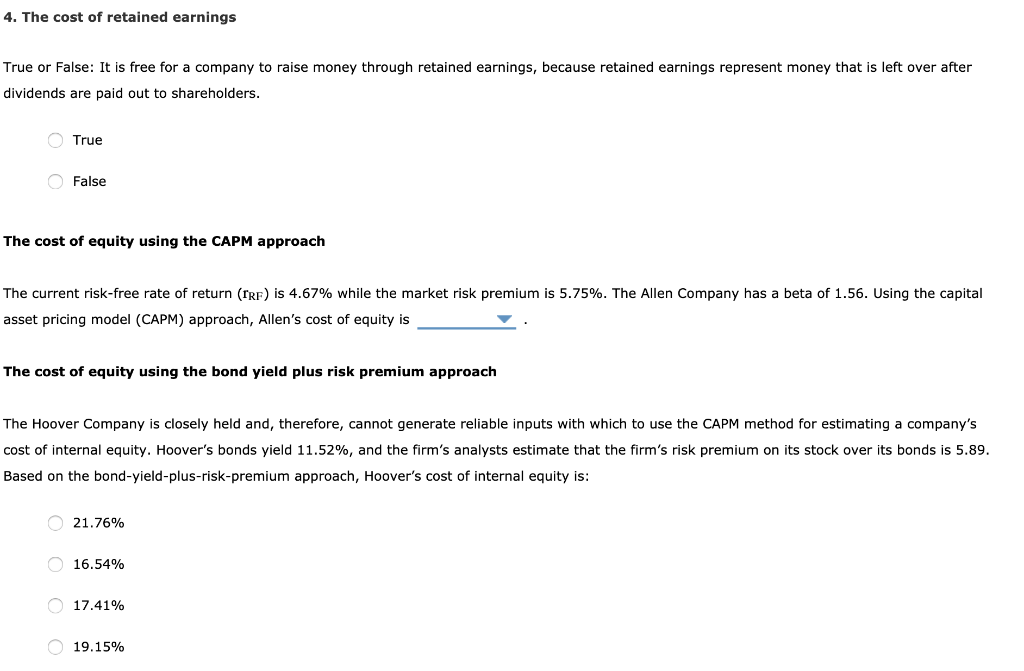4. The cost of retained earnings True or False: It is free for a company to raise money through retained earnings, because retained earnings represent money that is left over after dividends are paid out to shareholders. True False The cost of equity using the CAPM approach The current risk-free rate of return (rrF) is 4.67% while the market risk premium is 5.75%. The Allen Company has a beta of 1.56. Using the capital asset pricing model (CAPM) approach, Allen's...

• ### please complete all parts to the question 4. The cost of retained earnings the required rate...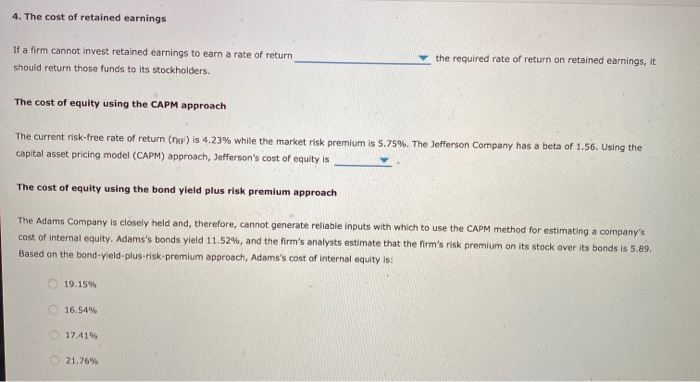please complete all parts to the question 4. The cost of retained earnings the required rate of return on retained earnings, it If a firm cannot invest retained earnings to earn a rate of return should return those funds to its stockholders, The cost of equity using the CAPM approach The current risk-free rate of return (TRF) is 4.23% while the market risk premium is 5.75%. The Jefferson Company has a beta of 1.56. Using the capital asset pricing model...

• ### 5. The cost of retained earnings Aa Aa If a firm cannot invest retained earnings to...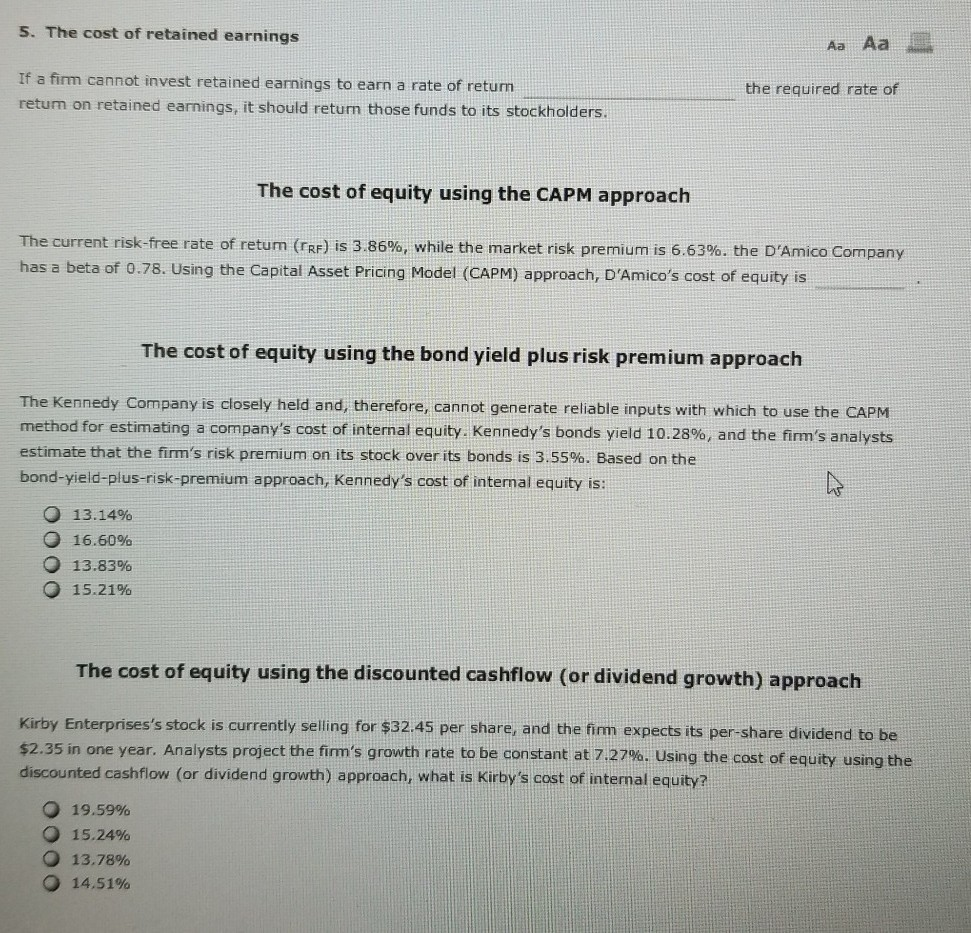5. The cost of retained earnings Aa Aa If a firm cannot invest retained earnings to earn a rate of return the required rate of return on retained earnings, it should return those funds to its stockholders. The cost of equity using the CAPM approach The current risk-free rate of return (rRE) is 3.86%, while the market risk premium is 6.63%. the D'Amico Company has a beta of 0.78. Using the Capital Asset Pricing Model (CAPM) approach, D'Amico's cost of...

• ### 4. The cost of retained earnings If a firm cannot invest retained earnings to earn a...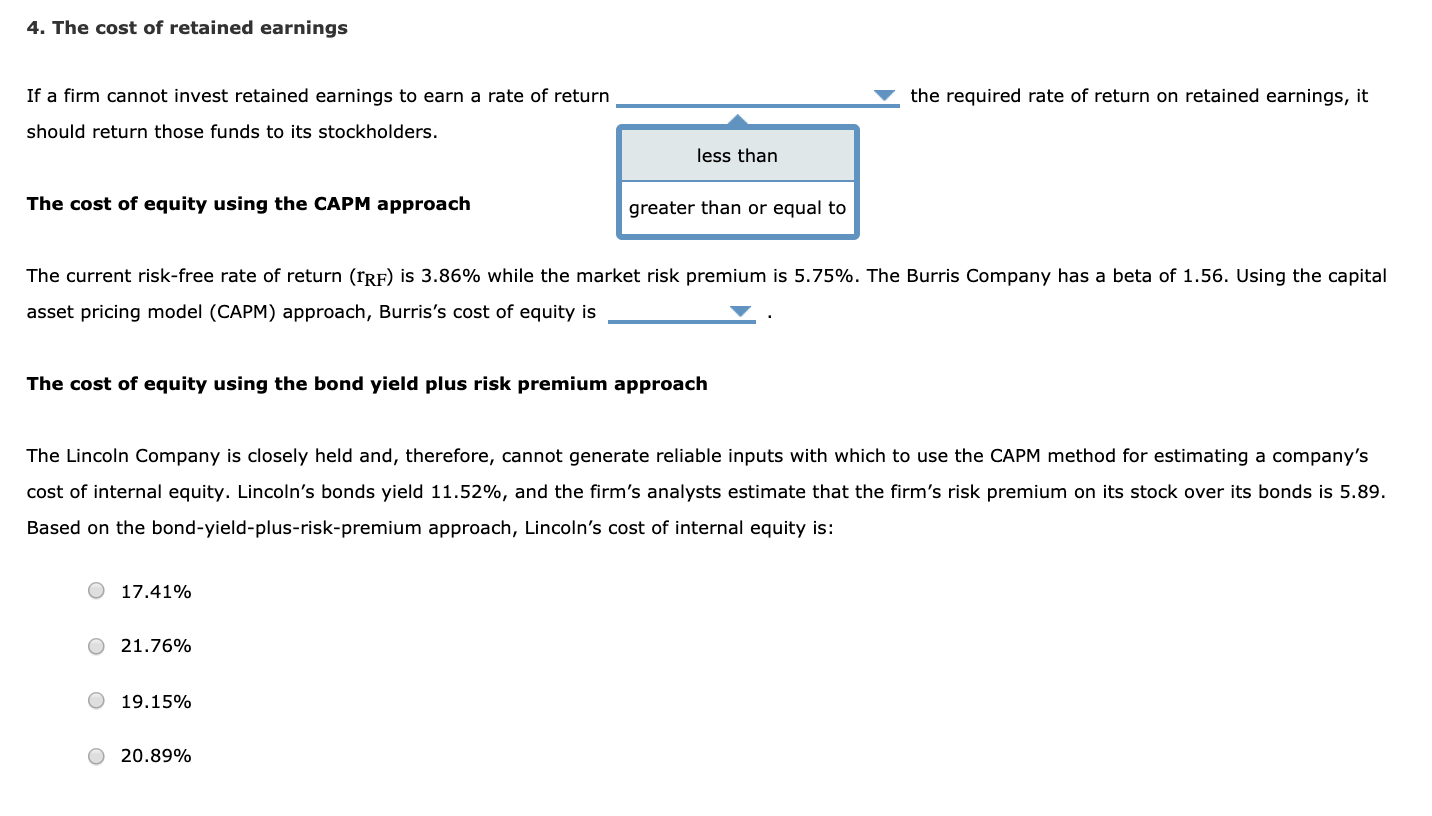4. The cost of retained earnings If a firm cannot invest retained earnings to earn a rate of return the required rate of return on retained earnings, it should return those funds to its stockholders. less than The cost of equity using the CAPM approach greater than or equal to The current risk-free rate of return (rrf) is 3.86% while the market risk premium is 5.75%. The Burris Company has a beta of 1.56. Using the capital asset pricing model...

Need Online Homework Help?